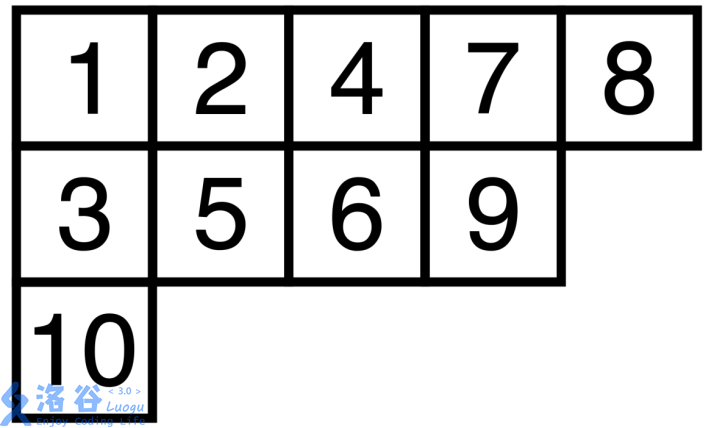（本文内容大量搬运自维基百科）$$\frac {n!}{\prod H(i,j)}$$

$$\frac {4!}{3\times2\times2\times1}=2$$

$$f_0=1,f_1=1,f_n=f_{n-1}+(n-1)f_{n-2}\ \ ,n\ge 2$$

Robinson–Schensted correspondence

• 检查当前行中是否有大于$j$的元素，如果没有，将$j$加入到这一行的末尾，结束这一过程。

• 否则用$j$替换掉当前行中第一个大于$j$的元素$k$，并将元素$k$按照一样的步骤从下一行开始插入

1 2 4 8 9
3 5 7 10
11

1 2 4 6 9
3 5 7 10
11

1 2 4 6 9
3 5 7 8
11

1 2 4 6 9
3 5 7 8
10

1 2 4 6 9
3 5 7 8
10
11

1 2 4 6 9
3 5 7 8
10
11
12

• 首先将$3$插入到第一个位置上，并且发现第一个位置有值是在第一个数插入的时候，在第二个杨氏矩阵的对应位置写上$1$。

$3$

$1$

• 之后将$8$插入，另一个表第一行第二个位置上写上$2$。

3 8

1 2
• 将$1$插入，这时候$3$的位置移到了第二行的第一列，这时候第二行第一列有值了，所以在第二个杨表里写上$3$。

1 8
3

1 2
3
• 插入$2$。

1 2
3 8

1 2
3 4
• 插入$4$

1 2 4
3 8

1 2 5
3 4
• 插入$7$。

1 2 4 7
3 8

1 2 5 6
3 4
• 插入$5$。

1 2 4 5
3 7
8

1 2 5 6
3 4
7
• 插入$6$。

1 2 4 5 6
3 7
8

1 2 5 6 8
3 4
7

$$\sum_{\lambda\in \mathcal{P}_n} (t_\lambda)^2=n!$$

（我当然不会证明）

Viennot's geometric construction